Next: Force Density Within Dielectric Up: Electrostatics in Dielectric Media Previous: Boundary Value Problems with

# Energy Density Within Dielectric Medium

Consider a system of free charges embedded in a dielectric medium. The increase in the total energy when a small amount of free charge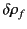is added to the system is given by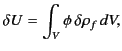(559)

where the integral is taken over all space, and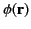is the electrostatic potential. Here, it is assumed that the original charges and the dielectric are held fixed, so that no mechanical work is performed. It follows from Equation (501) that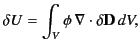(560)

where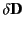is the change in the electric displacement associated with the charge increment. Now, the above equation can also be written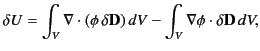(561)

giving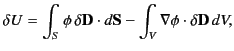(562)

where use has been made of the divergence theorem. If the dielectric medium is of finite spatial extent then we can neglect the surface term to give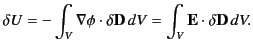(563)

This energy increment cannot be integrated unless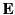is a known function of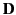. Let us adopt the conventional approach, and assume that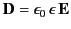, where the dielectric constant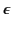is independent of the electric field. The change in energy associated with taking the displacement field from zero to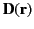at all points in space is given by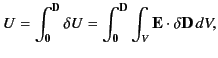(564)

or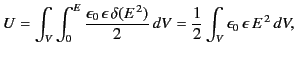(565)

which reduces to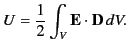(566)

Thus, the electrostatic energy density inside a dielectric is given by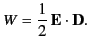(567)

This is a standard result that is often quoted in textbooks. Nevertheless, it is important to realize that the above formula is only valid in dielectric media in which the electric displacement,, varies linearly with the electric field,.Next: Force Density Within Dielectric Up: Electrostatics in Dielectric Media Previous: Boundary Value Problems with
Richard Fitzpatrick 2014-06-27